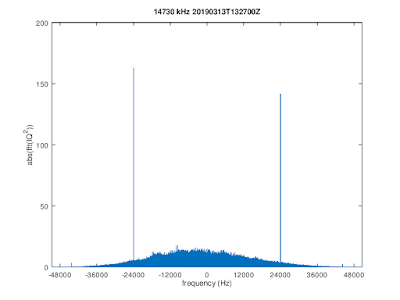## Thursday, March 21, 2019

### Interesting MSK-modulated signals on HF

Recently, a number of MSK-modulated signals with bandwidth ≈48 kHz were picked up on various KiwiSDRs on frequencies including 6840, 7730, 9490, 10640, 10840, 14730, 14780, and 14830 kHz. For some of these signals a TDoA analysis has been performed, pointing to a location close to Chicago:TDoA
Since the bandwidth of theses signals exceeds the available bandwidth of a single KiwiSDR channel, three recordings spaced 15 kHz were coherently combined using gr-kiwisdr.

The power spectrum of FFT(IQ2) shows two clear peaks at ±24kHz, so these signals are MSK modulated with 48,000 baud data rate.FFT(IQ2) showing two clear peaks at ±baud/2 → MSK modulation

For the coherently combined recording, the MSK demodulation quality plots shown below indicate that the quality is sufficient for extracting bit streams. These and the following plots were made with Octave code based on signal-analysis.MSK demodulation quality plots

Using a coherent demodulation technique the "X" and "Y" bit streams were obtained, where the "X" bits are modulated onto cos(t) and "Y" onto sin(t). The data rate for both "X" and "Y" is 24,000 bits/sec.

The autocorrelations of the "X" and "Y" bit streams do not reveal any frame structure, i.e., "X" and "Y" look like streams of perfect random bits. However, it turns out that the "Y" bit stream is entirely made up by a pseudo-random sequence generated by a LFSR, so this presumably is how the start locations of frames can be recovered from the received signal.

The taps for the length-20 LFSR generating the "Y" bit stream are
T = ,
where the LFSR taps T are defined such that the following property holds for a given bit sequence b
b(i) == mod(sum(b(i-20:i-1) .* T), 2)

The period of this LFSR was determined by simulating 2M bits using the found taps and a start state taken from the data. It is N = 917,497 = (23-1)×(217-1). The fact that N factorizes indicates that the polynomial in GF(2) defined by T is not prime, and indeed:
[20,19,17,16,15,13,12,10,9,7,6,4,3,1,0] = [3,1,0] × [17,16,15,14,13,12,10,8,5,4,0].
A valid start state S which generates "Y" is
S = [0,0,0,0,0,0,0,0,0,0,0,0,0,0,0,0,0,0,0,1].The ~"Y" bit stream is generated by a LFSR with 20 taps.

No structures have yet been found for the "X" bit stream which presumably carries data.